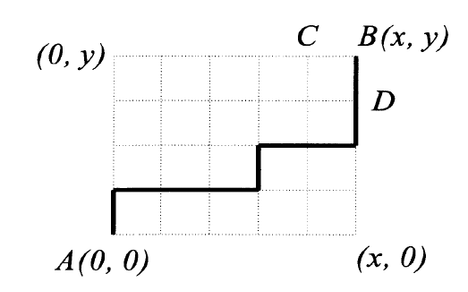# Third Dimensionridowan007SUST Intra University Pro...
Limits 2s, 512 MB

Mr. J wants to learn about combinatorics. He started reading a book on combinatorics and came across this problem:

A town, rectangular in shape, has a grid-like street network. It consists of x+1 parallel lines headed north-south and y+1 parallel lines headed east-west. You can think of Manhattan as an example. In how many ways can a car reach the north-east corner if it starts in the south-west comer and travels only in the east and north directions?"So the problem is, you are at $A$ $(0, 0)$ position in a grid. You can only go to the right or towards the top. You have to determine, how many ways you can go to $B$ $(x, y)$.

Now Mr. J found the solution of the problem in the book, and he started to wonder what if he adds a third dimension to this problem.

Imagine there is a box with $x$ width, $y$ height and $z$ depth. You are standing in one corner $(0, 0, 0)$. In each move, you can only go front (+1 in X dimension), right (+1 in Y dimension) and towards the top (+1 in Z dimension). So from $(A, B, C)$ you can go to $(A+1, B, C)$, $(A, B+1, C)$, or $(A, B, C+1)$ in one move.

Now determine how many ways you can go to the corner $(x, y, z)$ from $(0, 0, 0)$. Two ways are considered different if after any one of the moves they are in a different position in the box.

## Input

The first line of the input is $T$ ($1 ≤ T ≤ 150$), the number of test cases. Then $T$ test cases follow.

Each test case has 3 integers $x$, $y$, $z$ ($1 ≤ x, y, z ≤ 5$) in a line. They represent the width, the height and the depth of the box respectively.

## Output

For each test case, print a line in "Case #K: R" format where K is the case number and R is the desired result.

## Sample

InputOutput
3
1 1 1
1 1 2
2 1 1

Case #1: 6
Case #2: 12
Case #3: 12uDebug

### Submit